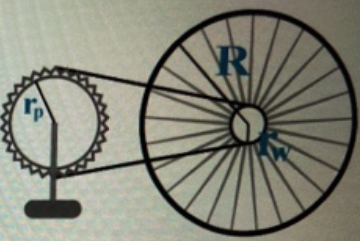# Problem: A bicyclist notes that the pedal sprocket has a radius of rp = 11 cm while the wheel sprocket has a radius of rw = 5.5 cm. The two sprockets are connected by a chain which rotates without slipping. The bicycle wheel has a radius R = 68 cm. When pedaling the cyclist notes that the pedal rotates at one revolution every t =  1.7s. When pedaling, the wheel sprocket and the wheel move at the same angular speed. Randomized Variables rp = 11 cmrw = 5.5 cmR = 68 cmt =  1.7sPart (a) The pedal sprocket and the wheel sprocket have the same__________.a) Angular and tangential speedb) Centripetal accelerationc) Tangential speed at their outer edgesd) RadiusPart (b) Calculate the angular speed of the pedal sprocket wp, in radians per second. Part (c) Calculate the linear speed of the outer edge of the pedal sprocket vp in centimeters per second. Part (d) Calculate the angular speed of the wheel sprocket ω w  in radians per second  Part (e) Calculate the linear speed of the bicycle v, in meters per second, assuming the wheel does not slip across the ground.Part (f) If the cyclist wanted to travel at a speed of v2 = 4.5 m/s, how much time, in seconds should elapse as the pedal makes one complete revolution?

###### FREE Expert Solution

Angular velocity:

$\overline{){\mathbf{\omega }}{\mathbf{=}}\frac{\mathbf{∆}\mathbf{\theta }}{\mathbf{∆}\mathbf{T}}}$

Tangential speed:

$\overline{){\mathbf{v}}{\mathbf{=}}{\mathbf{r}}{\mathbf{\omega }}}$

Part (a)

Radius is different. Chian speed (Tangential speed) is the same for both. Angular velocity is different because of the difference in radius.

83% (155 ratings)###### Problem Details

A bicyclist notes that the pedal sprocket has a radius of r= 11 cm while the wheel sprocket has a radius of rw = 5.5 cm. The two sprockets are connected by a chain which rotates without slipping. The bicycle wheel has a radius R = 68 cm. When pedaling the cyclist notes that the pedal rotates at one revolution every t =  1.7s. When pedaling, the wheel sprocket and the wheel move at the same angular speed.

Randomized Variables

r= 11 cm

rw = 5.5 cm

R = 68 cm

t =  1.7sPart (a) The pedal sprocket and the wheel sprocket have the same__________.

a) Angular and tangential speed

b) Centripetal acceleration

c) Tangential speed at their outer edges

Part (b) Calculate the angular speed of the pedal sprocket wp, in radians per second.

Part (c) Calculate the linear speed of the outer edge of the pedal sprocket vp in centimeters per second.

Part (d) Calculate the angular speed of the wheel sprocket ω w  in radians per second

Part (e) Calculate the linear speed of the bicycle v, in meters per second, assuming the wheel does not slip across the ground.

Part (f) If the cyclist wanted to travel at a speed of v= 4.5 m/s, how much time, in seconds should elapse as the pedal makes one complete revolution?